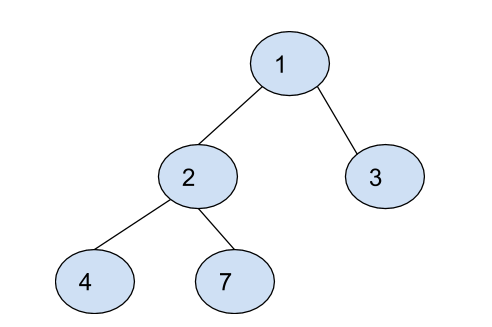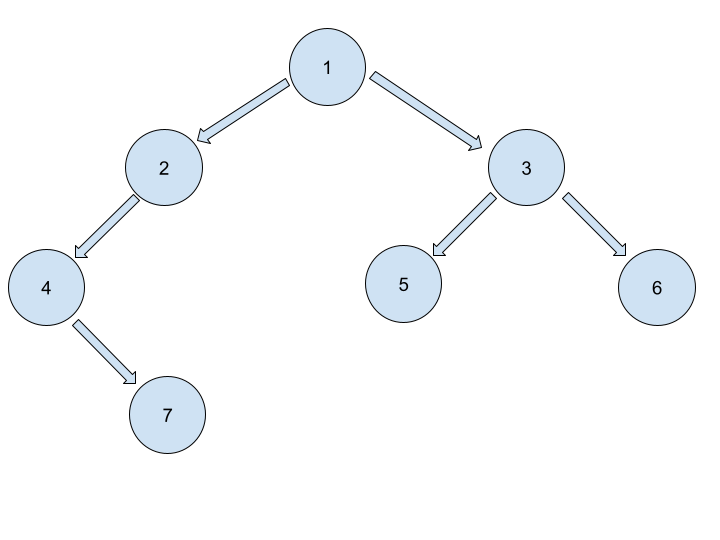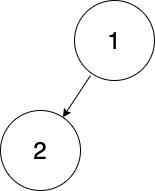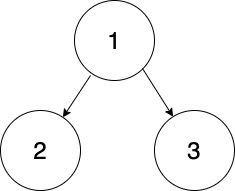Problem title
Difficulty
Avg time to solve

Palindromic Substrings
Moderate
20 mins
Sum Between Zeroes
Easy
20 mins
Longest Substring Without Repeating Characters
Moderate
20 mins
Contains Duplicate ll
Moderate
35 mins
Rearrange The Array
Moderate
15 mins
Trailing Zeros in Factorial
Moderate
15 mins
Maximum Size Rectangle Sub-matrix With All 1's
Hard
10 mins
Easy
15 mins
Construct Binary Tree From Inorder and Preorder Traversal
Easy
10 mins
Check If Binary Representation of a Number is Palindrome
Easy
15 mins18

# Construct Binary Tree From Inorder and Preorder Traversal

Difficulty: EASY
Avg. time to solve
10 min
Success Rate
90%

Problem Statement

#### You have been given the preorder and inorder traversal of a binary tree. Your task is to construct a binary tree using the given inorder and preorder traversals.

##### Note:
``````You may assume that duplicates do not exist in the given traversals.
``````
##### For example :
``````For the preorder sequence = [1, 2, 4, 7, 3] and the inorder sequence = [4, 2, 7, 1, 3], we get the following binary tree.
``````##### Input Format:
``````The first line contains an integer 'T' which denotes the number of test cases or queries to be run. Then the test cases are as follows.

The first line of each test case contains an integer ‘N’ denoting the number of nodes in the binary tree.

The second line of each test case contains ‘N’ integers denoting the preorder traversal of the binary tree.

The third line of each test case contains ‘N’ integers denoting the inorder traversal of the binary tree.
``````
##### Output Format:
``````For each test case, print the level order traversal of the constructed binary tree separated by a single-space.

For example, the output for the tree depicted in the below image would be :
````````````Level Order Traversal:
1
2 3
4 5 6
7

Explanation :
Level 1 :
The root node of the tree is 1

Level 2 :
Left child of 1 = 2
Right child of 1 = 3

Level 3 :
Left child of 2 = 4
Right child of 2 = null
Left child of 3 = 5
Right child of 3 = 6

Level 4 :
Left child of 4 = null
Right child of 4 = 7
Left child of 5 = null
Right child of 5 = null
Left child of 6 = null
Right child of 6 = null

Level 5 :
Left child of 7 = null
Right child of 7 = null
``````
##### Note :
``````Here, if the node is null, print nothing. The above format was just to provide clarity on how the output is formed for a given tree.
The sequence will be put together in a single line separated by a single space. Hence, for the above-depicted tree, the output will be:

1 2 3 4 5 6 7

The output of each test case will be printed in a separate line.
``````
##### Note :
``````You do not need to print anything; it has already been taken care of. You just need to return the root node of the constructed binary tree.
``````
##### Constraints:
``````1 <= T <= 100
1 <= N <= 3000
1 <= data <= 10^4

Where ‘T’ is the number of test cases, and ‘N’ is the total number of nodes in the binary tree, and “data” is the value of the binary tree node.

Time Limit: 1sec
``````
##### Follow-up:
``````Can you solve this in O(N) time complexity?
``````
##### Sample Input 1:
``````3
5
1 2 4 7 3
4 2 7 1 3
2
1 2
2 1
3
1 2 3
2 1 3
``````
##### Sample Output 1:
``````1 2 3 4 7
1 2
1 2 3
``````
##### Explanation of Sample Input 1:
``````For the first test case, the tree after the construction is shown below.
````````````For the second test case, the level order of the constructed tree is [1, 2]. The tree after the construction is shown below.
````````````For the third test case, the level order of the constructed tree is [1, 2, 3]. The tree after the construction is shown below.
``````##### Sample Input 2:
``````2
3
1 2 3
3 2 1
1
7
7
``````
##### Sample Output 2:
``````1 2 3
7
``````Console